2019-04-11 11:01:34 hxf19961219 阅读数 969

a.介绍

a.数据集分布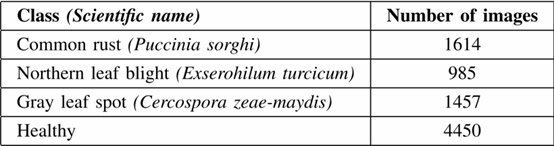b.网络架构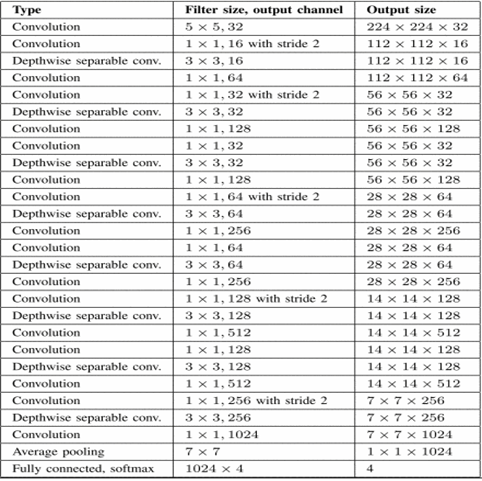c.实验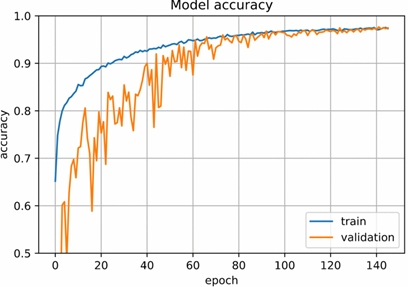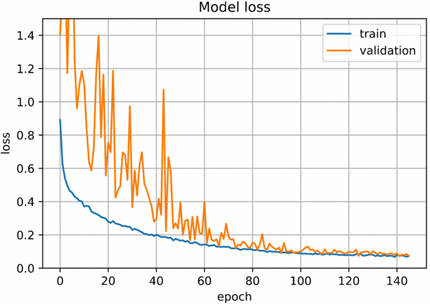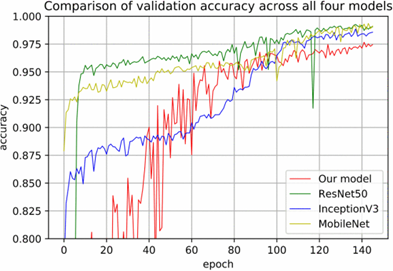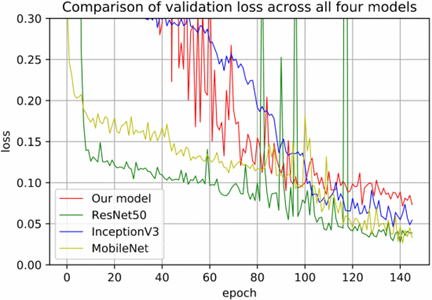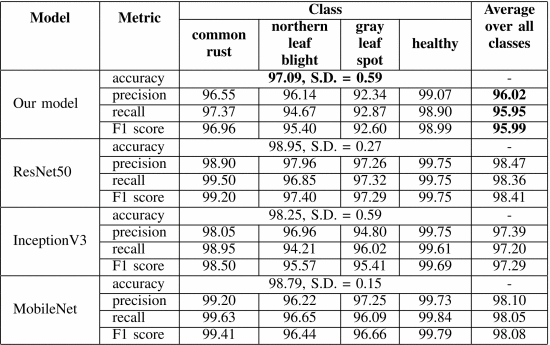d.结论

2020-03-24 14:00:07 hxf19961219 阅读数 119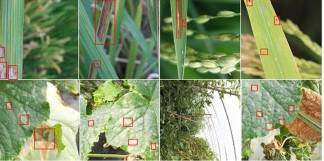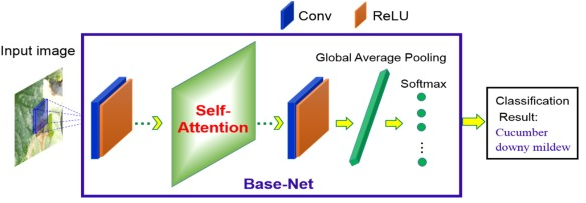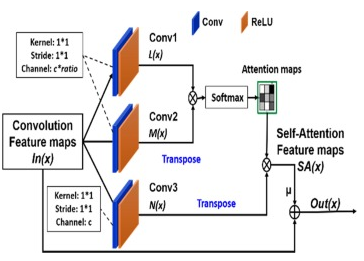2020-02-15 21:56:35 bless2015 阅读数 45

## 卷积神经网络（Convolutional Neural Networks, CNN）

### 前言

ImageNet图像识别挑战赛（ImageNet Large Scale Visual Recognition Challenge），在2012年之前参赛模型普遍选用特征+支持向量机（Support Vector Machine，SVM）。在2012年，Alex Krizhevsky和他的导师Geoff Hinton提出的基于卷积神经网络架构的模型，以巨大的优势获得当年的ImageNet图像分类挑战赛的桂冠。$H=f({\sum_{i=1}^n}x_iw_{ij}+b_h)$### 局部感知野### 卷积神经网络结构LeNet-5模型一共有7层。有三个卷基层：C1，C3，C5。
C1层有6个55大小的卷积核，可以提取到6个2828大小的特征图（feather map）。这一层可训练的参数为156个，每个滤波器为$5*5$个参数，加上一个bias参数，有6个滤波器。有156个参数。一共有1562828=122304个连接。
C3层包括1500权重和16个偏置，有1516个训练参数和151600个连接。
S2层和C3层之间不是全连接Lecun设计了如上图所示的连接方式，不仅减少了权重数量，而且还相互组合由C3提取特征，破坏了网络的对称性。
C5层包含120个feather map，尺寸为$1*1$
LeNet-5模型还包括两个下采样层S2，S4。S2包括6个特征图，特征图的每个单元与上一层$2*2$大小的区域连接。S2层每个单元4个参数相加，乘以一个可训练参数加一个可训练偏置作为输出，所以一共有$12（6*(1+1)=12）$个可训练参数。每个特征图都与C1中2*2的区域和1个偏置相连接，所以一共有$5880（6*5*14*14=5880）$个连接。同理，S4有32个可训练参数和156000个连接。

$x_j^l=\beta_j^ldown(x_j^{l-1})+b_j^l$
down()函数是采样方式，有平均池化（就是取被池化区域像素的平均值），最大采样（就是取被池化区域像素的最大值）

### 卷积神经网络的训练$z={\sum_{i=1}^nXW+b}$
$a=f(z)$
$J=loss(z)$

${\{(x_1,y_1)，(x_2,y_2)……(x_n,y_n)\}} （说明：x和y都是向量）$

$loss = \frac{1}{2} (\acute{y^i}-y^i)^2$

$\frac{∂J}{∂w}=\frac{∂J}{∂a}\frac{∂a}{∂z}\frac{∂z}{∂w}$
$\frac{∂J}{∂b}=\frac{∂J}{∂a}\frac{∂a}{∂z}\frac{∂z}{∂b}$### 多通道

#### 多通道输入## 实现

### 手写实现

Y = torch.zeros(6, 7)
X[:, 2: 6] = 0
Y[:, 1] = 1
Y[:, 5] = -1
print(X)
print(Y)


tensor([[1., 1., 0., 0., 0., 0., 1., 1.],
[1., 1., 0., 0., 0., 0., 1., 1.],
[1., 1., 0., 0., 0., 0., 1., 1.],
[1., 1., 0., 0., 0., 0., 1., 1.],
[1., 1., 0., 0., 0., 0., 1., 1.],
[1., 1., 0., 0., 0., 0., 1., 1.]])
tensor([[ 0., 1., 0., 0., 0., -1., 0.],
[ 0., 1., 0., 0., 0., -1., 0.],
[ 0., 1., 0., 0., 0., -1., 0.],
[ 0., 1., 0., 0., 0., -1., 0.],
[ 0., 1., 0., 0., 0., -1., 0.],
[ 0., 1., 0., 0., 0., -1., 0.]])

import torch
import torch.nn as nn

def corr2d(X, K):
H, W = X.shape
h, w = K.shape
Y = torch.zeros(H - h + 1, W - w + 1)
for i in range(Y.shape):
for j in range(Y.shape):
Y[i, j] = (X[i: i + h, j: j + w] * K).sum()
return Y
X = torch.tensor([[0, 1, 2], [3, 4, 5], [6, 7, 8]])
K = torch.tensor([[0, 1], [2, 3]])
Y = corr2d(X, K)
print(Y)


tensor([[19., 25.],
[37., 43.]])

class Conv2D(nn.Module):
def __init__(self, kernel_size):
super(Conv2D, self).__init__()
# 随机初始化
self.weight = nn.Parameter(torch.randn(kernel_size))
self.bias = nn.Parameter(torch.randn(1))

def forward(self, x):
# 卷积+偏置
return corr2d(x, self.weight) + self.bias


conv2d = Conv2D(kernel_size=(1, 2))
step = 30
lr = 0.01
for i in range(step):
Y_hat = conv2d(X)
l = ((Y_hat - Y) ** 2).sum()
l.backward()
# 梯度下降

# 梯度清零
if (i + 1) % 5 == 0:
print('Step %d, loss %.3f' % (i + 1, l.item()))

print(conv2d.weight.data)
print(conv2d.bias.data)


Step 5, loss 4.569
Step 10, loss 0.949
Step 15, loss 0.228
Step 20, loss 0.060
Step 25, loss 0.016
Step 30, loss 0.004
tensor([[ 1.0161, -1.0177]])
tensor([0.0009])

### pytorch实现

#### 卷积层的简洁实现

in_channels (python:int) – Number of channels in the input imag
out_channels (python:int) – Number of channels produced by the convolution
kernel_size (python:int or tuple) – Size of the convolving kernel
stride (python:int or tuple, optional) – Stride of the convolution. Default: 1
bias (bool, optional) – If True, adds a learnable bias to the output. Default: True

X = torch.rand(4, 2, 3, 5)
print(X.shape)

conv2d = nn.Conv2d(in_channels=2, out_channels=3, kernel_size=(3, 5), stride=1, padding=(1, 2))
Y = conv2d(X)
print('Y.shape: ', Y.shape)
print('weight.shape: ', conv2d.weight.shape)
print('bias.shape: ', conv2d.bias.shape)


torch.Size([4, 2, 3, 5])
Y.shape: torch.Size([4, 3, 3, 5])
weight.shape: torch.Size([3, 2, 3, 5])
bias.shape: torch.Size()

#### 池化层的简洁实现

kernel_size – the size of the window to take a max over
stride – the stride of the window. Default value is kernel_size

X = torch.arange(32, dtype=torch.float32).view(1, 2, 4, 4)
pool2d = nn.MaxPool2d(kernel_size=3, padding=1, stride=(2, 1))
Y = pool2d(X)
print(X)
print(Y)


tensor([[[[ 0., 1., 2., 3.],
[ 4., 5., 6., 7.],
[ 8., 9., 10., 11.],
[12., 13., 14., 15.]],
[[16., 17., 18., 19.],
[20., 21., 22., 23.],
[24., 25., 26., 27.],
[28., 29., 30., 31.]]]])
tensor([[[[ 5., 6., 7., 7.],
[13., 14., 15., 15.]],
[[21., 22., 23., 23.],
[29., 30., 31., 31.]]]])

2019-08-21 15:47:16 j_starry 阅读数 214

……

……

### 3 Materials and Methods

3.1数据集。从训练阶段到评价识别算法的性能，目标识别研究的各个阶段都需要合适的数据集。为数据集收集的所有图像都是从互联网上下载的，通过疾病和植物名称在不同的来源上搜索，这些来源来自不同的语言，如拉丁语、英语、德语、塞尔维亚语和匈牙利语。所有图片被分成15个不同的类。十三个类别代表了植物病害，这些病害可以从叶子上直观地判断出来。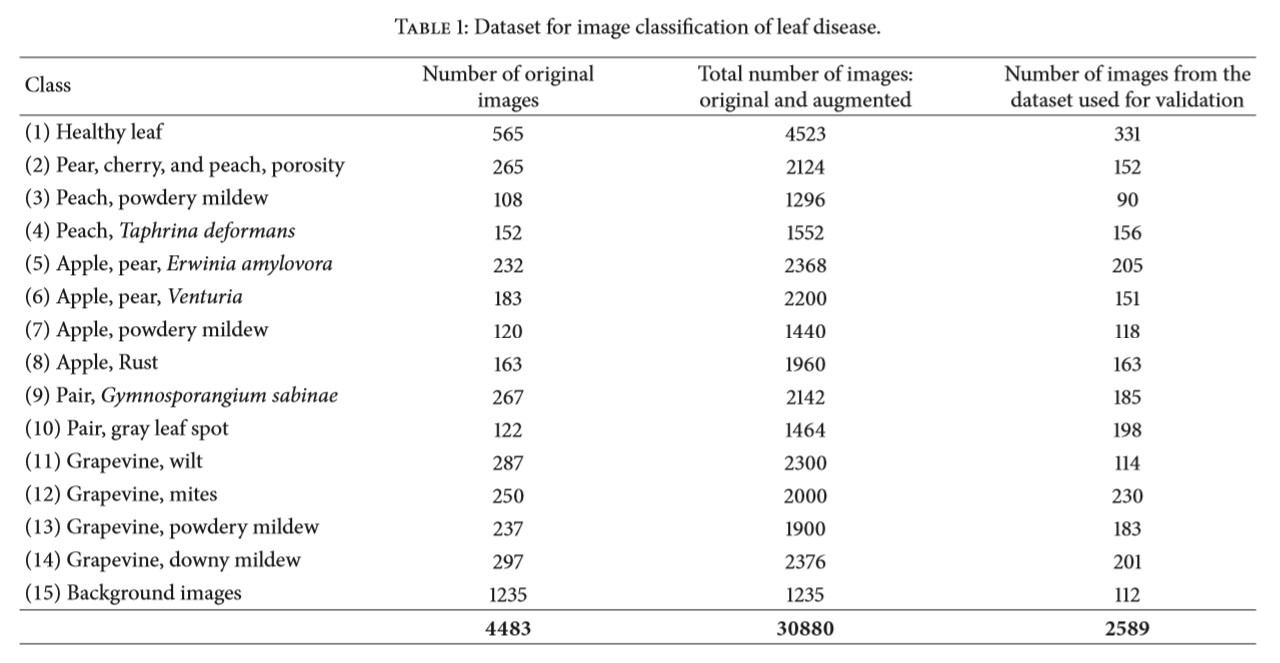3.2图像预处理和标签。图片是从互联网上下载的，具有不同的分辨率和质量。为了获得更好的特征提取，我们对作为深度神经网络分类器数据集的最终图像进行预处理，以获得一致性。此外，图像预处理的过程包括手工裁剪所有图像，在叶子周围做一个正方形，以突出感兴趣的区域(植物叶子)。在数据集图像采集阶段，分辨率较小、尺寸小于500px的图像不被认为是数据集的有效图像。此外，只有感兴趣的区域分辨率更高的图像才被标记为数据集的合格候选。这样，就确保了图像包含了学习特征所需的所有信息。该数据集使用的图像大小为256×256，以减少训练时间，训练时使用python和OpenCV框架自动计算。

3.3增强的过程。应用增强的主要目的是增加数据集，对图像进行轻微的失真，从而减少训练阶段的过拟合。在机器学习和统计学中，当统计模型描述随机噪声或错误而不是潜在关系时，就会出现过拟合。图像增强包含几种转换技术之一，包括仿射转换、透视转换和简单的图像旋转。使用仿射变换来表示平移和旋转，其中原始图像中的所有平行线在输出图像中仍然是平行的。要找到一个变换矩阵，需要在输出图像的相应位置加上原图像中的三点。透视变换需要a3×3变换矩阵。即使在转型之后，直线仍然是直线。在增强过程中，采用了简单的图像旋转，以及不同轴对称度的旋转。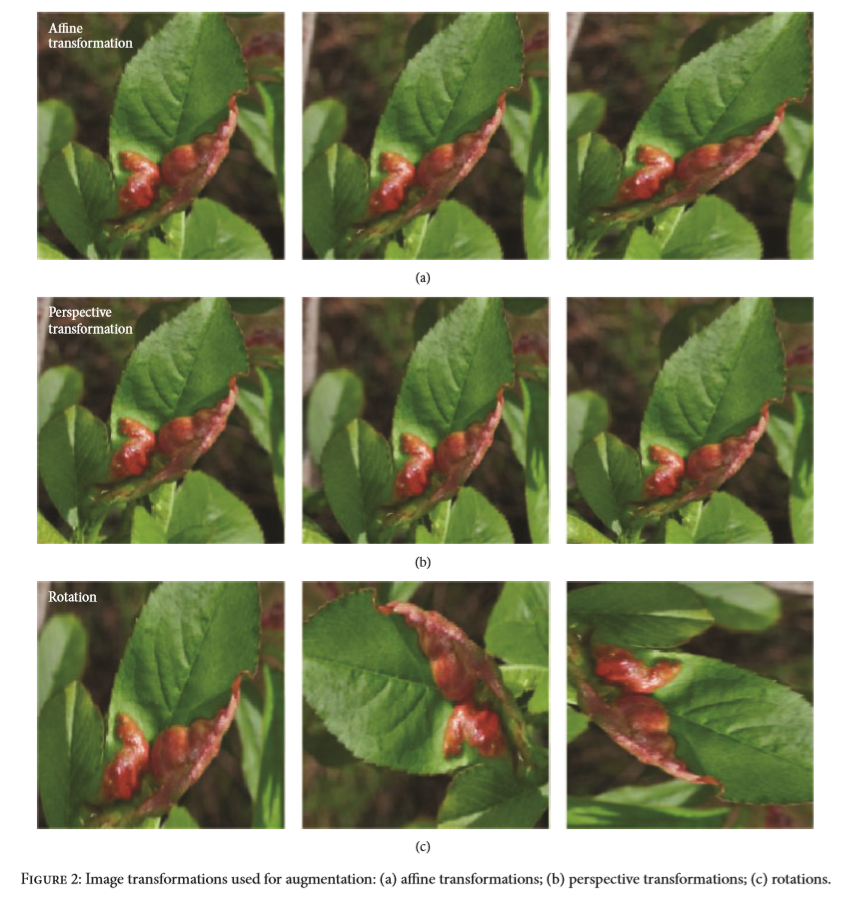3.4神经网络的训练。提出了利用3.1节描述的数据集训练深度卷积神经网络建立图像分类模型。有几个著名的最先进的深度学习框架，如theano和Lua、Torch7的机器学习库。此外，还有Caffe，这是一个开源的深度学习框架，由bvlc开发，其中包含了预先训练好的CaffeNet模型。为了本研究，我们使用了这个框架，以及一组平均大数据集(ImageNet)的权值。

Caffe框架既适合于研究实验，也适合于行业部署。框架的核心是C++，并提供命令行、Python和matlab接口。Caffe与cudnn库的集成加速了Caffe模型的开发[41,42]。CaffeNet是一个深度CNN，它有多个层，可以从输入图像逐步计算特性。具体来说，该网络包括8个学习层、5个卷积层和3个全连接层。

CaffeNet体系结构被认为是一个起点，但是修改和调整了它来支持15个类别(类)。最后一层进行了修改，并将softmax层的输出参数化，以满足本研究的要求。

……

CNN和ReLUs的训练速度要快好几倍。该方法适用于所有卷积和全连接层的输出。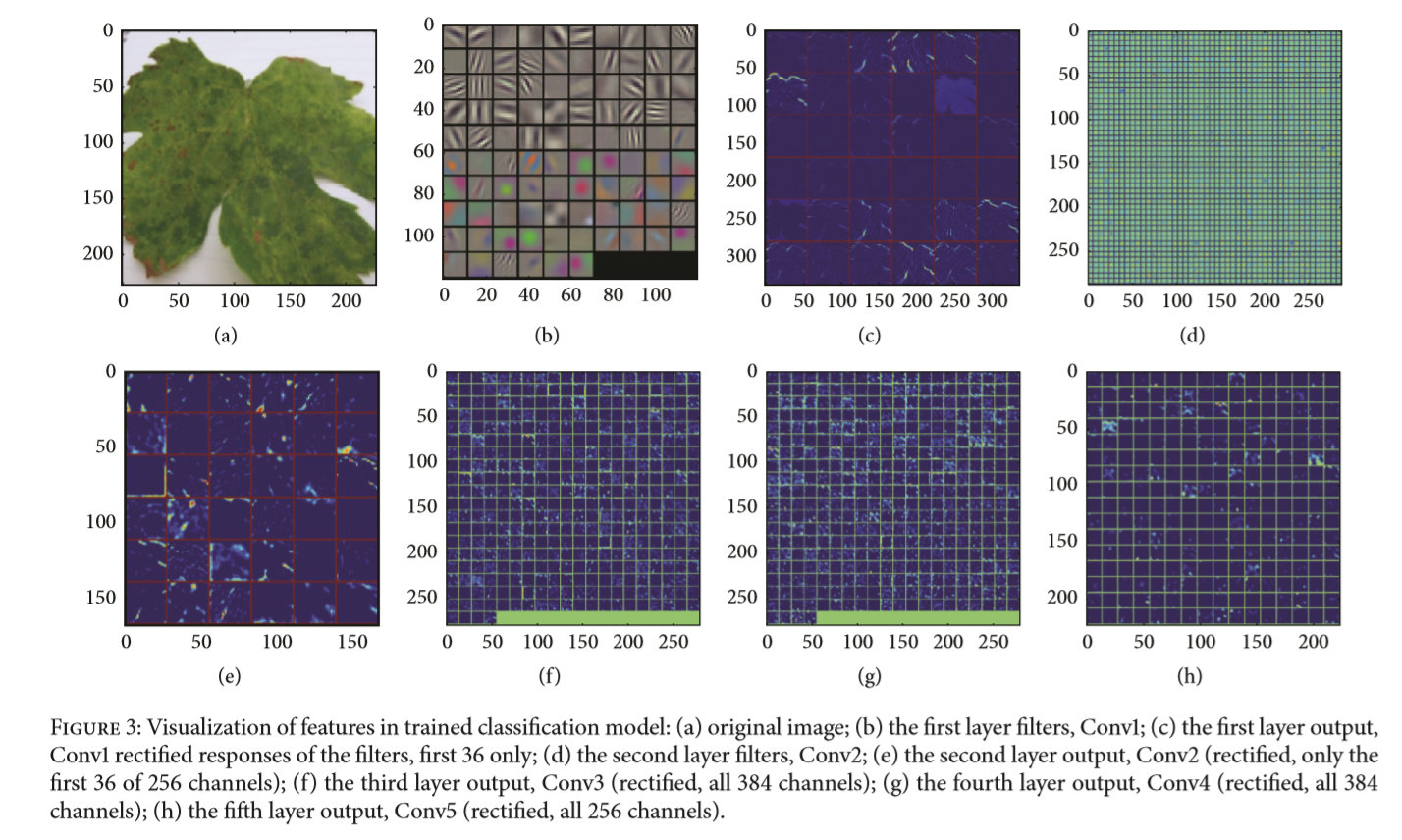CNNs的另一个重要层是池化层，它是非线性下采样的一种形式。池化操作给出了平移不变性的形式;它依赖于输入的每个深度切片，并在空间上调整其大小。重叠池化有利于减少过度拟合

相对于传统的no-overlapping pooling，采用Overlapping Pooling不仅可以提升预测精度，同时一定程度上可以减缓过拟合。
相比于正常池化（步长s=2，窗口z=2） 重叠池化(步长s=2，窗口z=3) 可以减少top-1, top-5分别为0.4% 和0.3%；重叠池化可以避免过拟合。）

CNNs的进步是由于它们能够学习丰富的中级图像表示，而不是使用手工设计的低层图像特征再通过其他图像分类方法来分类。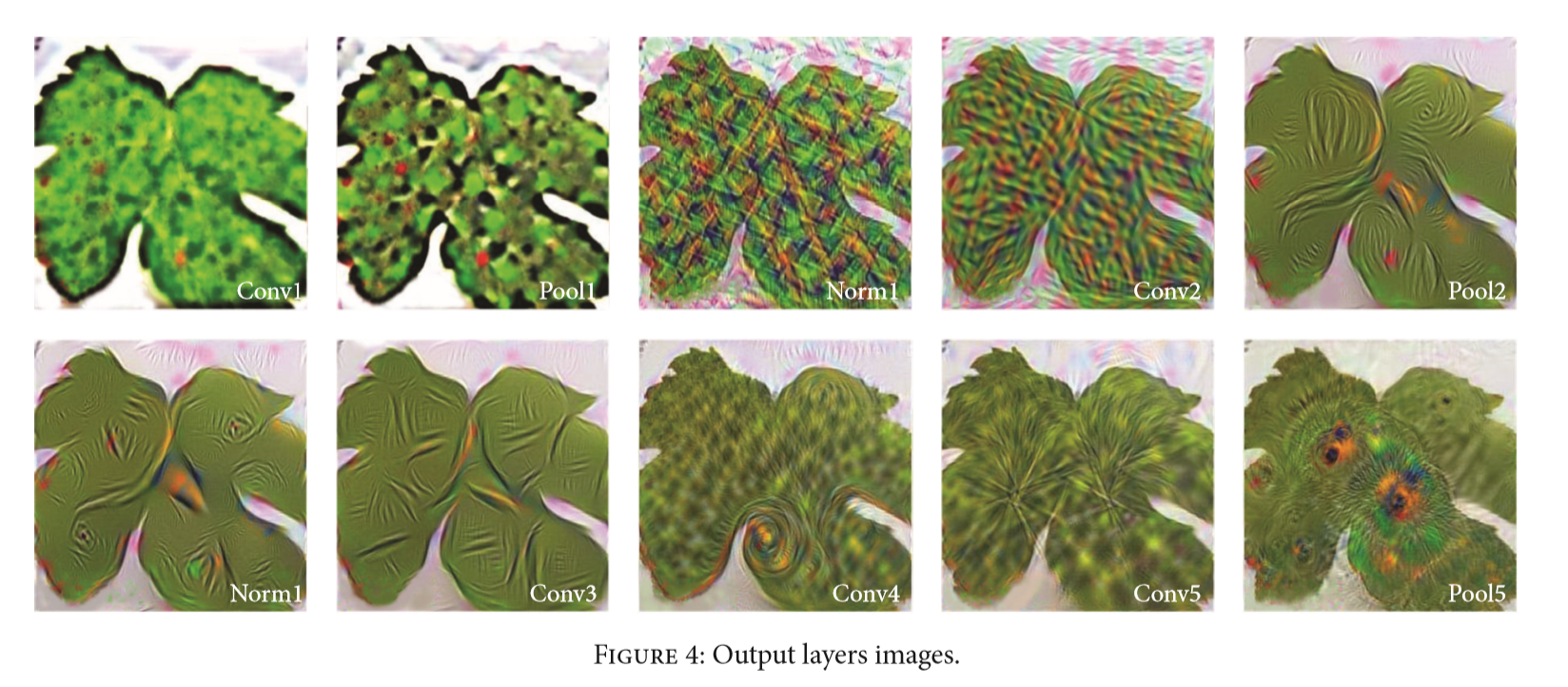3.5执行测试。测量人工神经网络性能的常用方法是将数据分离到训练集和测试集，然后在训练集上训练一个神经网络，并使用测试集进行预测。因此，由于测试集的原始输出值和我们预测的模型输出值是已知的，因此我们的预测精度可以计算出来。训练时使用30880张图像，使用2589张图像进行测试。

3.6.Fine-Tuning。微调是通过对过程或功能进行小的修改来提高或优化结果，从而提高过程或功能的有效性或效率。原始的caffeNet模型的分类功能是通过softmax计算imagenet中1000个类的概率来分类的。微调的学习实验需要一些学习，但它们仍然比从零开始学习快得多。为了开始微调过程，删除了这个softmax分类器，如第3.4节所述，并进行了说明，然后随机初始化值。利用3.1节所述数据集中的数据，采用反向传播算法对新的softmax分类器进行从无到有的训练。这个数据集有15个不同的类别。由于本研究使用的数据与ImageNet (ILSVRC-2012)相比较小，对微调隐藏层使用较低的初始学习率限制了过度拟合。顶层的学习率设置为10的，而其他7个学习层的学习率设置为0.1。反向传播算法运行了100,000次迭代。微调过程是隐层参数和超参数不断变化的过程。通过参数的实验调整，得到了最适合植物病害检测的模型。第四节给出了模型微调的结果，并进行了进一步的解释。

3.7设备。采用单台PC机对描述的植物病害检测模型进行了全过程的训练和测试。在图形处理单元(GPU)模式下对fcns进行训练。每一次训练都要花大约8个小时在这台机器上。

### 4. Results and Discussion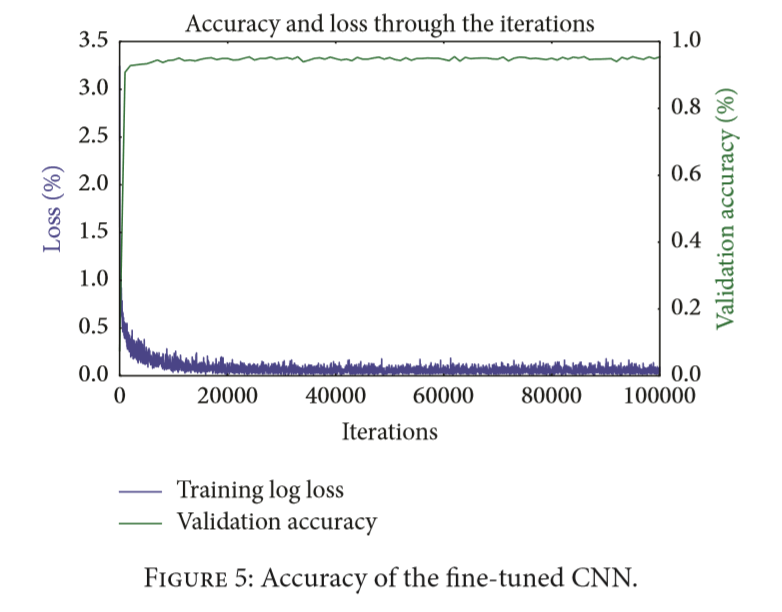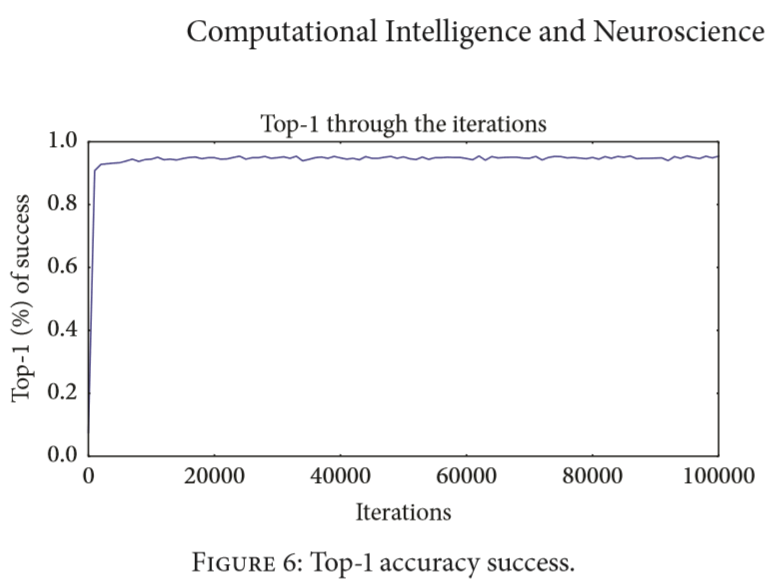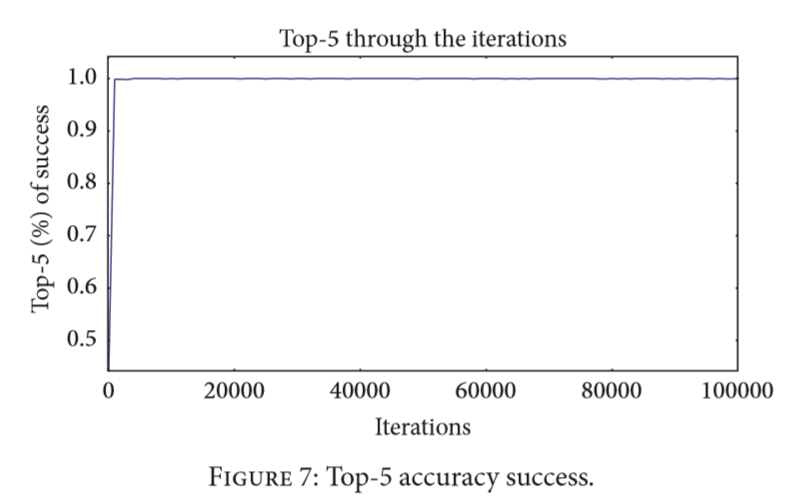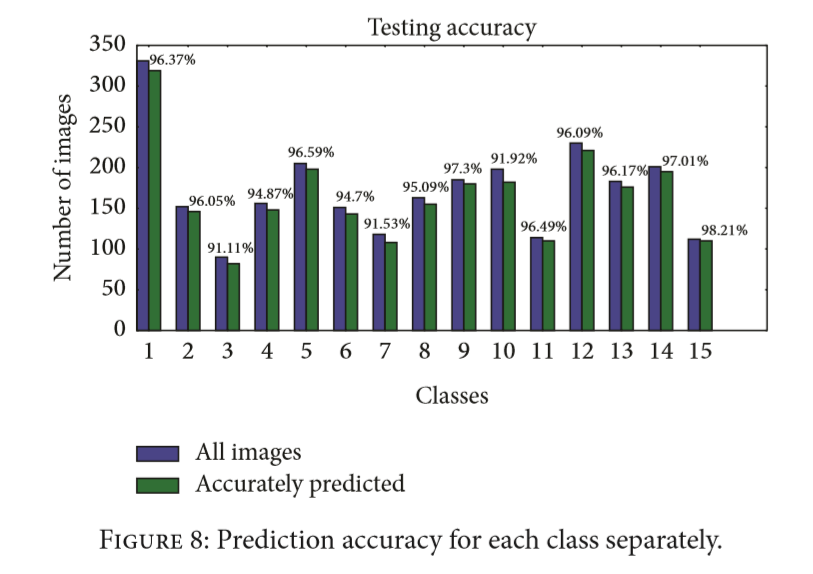### 5. Conclusions

2018-01-08 11:03:00 weixin_34161083 阅读数 81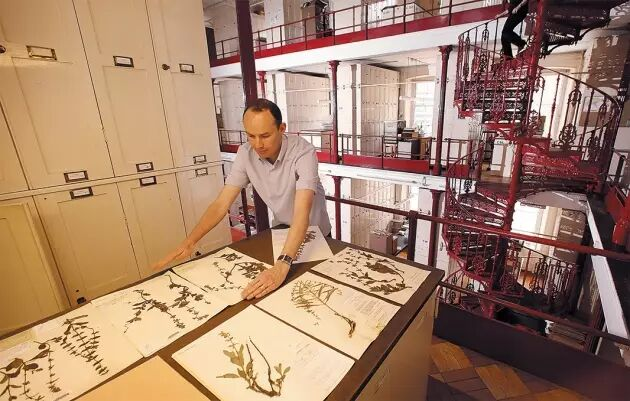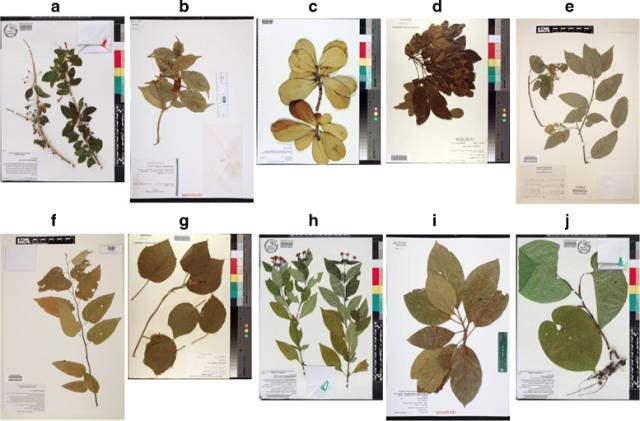数字化的植物标本打开了研究人员从世界各地收集标本的新世界

https://bmcevolbiol.biomedcentral.com/articles/10.1186/s12862-017-1014-z

—— ——